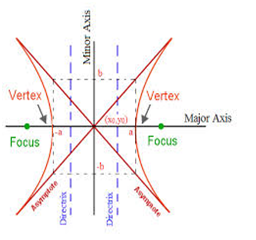Click to Chat

1800-1023-196

+91-120-4616500

CART 0

• 0

MY CART (5)

Use Coupon: CART20 and get 20% off on all online Study Material

ITEM
DETAILS
MRP
DISCOUNT
FINAL PRICE
Total Price: Rs.

There are no items in this cart.
Continue Shopping• Complete JEE Main/Advanced Course and Test Series
• OFFERED PRICE: Rs. 15,900
• View Details

```Revision Notes on Hyperbola

The standard equation of hyperbola with reference to its principal axis along the coordinate axis is given by x2/a2 - y2/b2 = 1, where b2 = a2 (e2 -1)

The foci of the hyperbola are S(ae, 0) and S’ = (-ae, 0)

Equations of the directrices are given by x = a/e and x = -a/e

The coordinates of vertices are A’ = (-a, 0) and A = (a,0)

The length of latus rectum is 2b2/a = 2a(e2 - 1)

The length of the transverse axis of the hyperbola is 2a.

The difference of the focal distances of any point on the hyperbola is constant and is equal to transverse axis.

x2/a2 - y2/b2 = 1 and -x2/a2 + y2/b2 = 1 are conjugate hyperbola of each other.

If e1 and e2are the eccentricities of the hyperbola and its conjugate then e1-2+ e2-2 = 1

The foci of a hyperbola and its conjugate are concyclic and form the vertices of a square.

The length of the transverse axis of a hyperbola is 2a and the transverse axis and conjugate axis together constitute the principal axis of the hyperbola.

Whether two hyperbolas are similar or not is decided on the basis of their eccentricity. The hyperbolas with same eccentricity are same.The eccentricity of rectangular hyperbola is √2 and the length of its latus rectum is equal to its transverse or conjugate axis.

The equation of the auxiliary circle of the hyperbola is given by x2+ y2 = a2

In parametric form, the equations x = a sec θand  y = b tan θ together represent the hyperbola x2/a2 - y2/b2 = 1. Here θ is a parameter.

The point P(x1, y1) lies within, on or outside the ellipse according as x12/a2 - y12/b2 = 1 is positive, zero or negative.

The line y = mx + c is a secant, a tangent or passes outside the hyperbola  x2/a2 - y2/b2 = 1 according as whether c2 is > = or < a2m2 - b2

Equations of tangents

Equation of tangent to hyperbola x2/a2 - y2/b2 = 1at the point (x1, y1) is xx1/a2 - yy1/b2 = 1

Equation of tangent to hyperbola x2/a2 - y2/b2 = 1at the point (a sec θ, b tan θ) is (x sec θ)/a - (y tan θ)/b= 1

y = mx ±[(a2m2- b2] can also be taken as the tangent to the hyperbola x2/a2 - y2/b2 = 1

Equations of normal

Equation of normal to the hyperbola x2/a2 - y2/b2 = 1 at the point (x1, y1) is a2 x/x1+ b2 y/y1 = a2 - b2 = a2e2

Equation of normal at the point P(a sec θ, b tan θ) on the hyperbola x2/a2 - y2/b2 = 1 is ax/sec θ + by/ tan θ = a2+b2 = a2e2

The equation of director circle is x2 + y2 = a2 - b2

The equations of the asymptotes of the hyperbola are x/a + y/b = 0 and x/a - y/b = 0. This can be combined as x2/a2 - y2/b2 = 0

The asymptotes of the hyperbola and its conjugate are same

The asymptotes pass through the center of the hyperbola and the bisectors of the angles between the asymptotes are the axis of a hyperbola

The equation xy = c2 represents a rectangular hyperbola

ü In a hyperbola b2 = a2 (e2 – 1). In the case of rectangular hyperbola (i.e., when b = a) result become a2 = a2(e2 – 1) or e2 = 2 or e =√2

ü In parametric form, its coordinates are x= ct, y = c/t, t ∈ R ~ {0}

ü Equation of a chord joining the points P(t1) and Q(t2) is

x + t1t2y = c(t1 + t2) with slope m = -1/ t1t2

Equation of tangent at P (x1, y1) is x/x1 + y/y1 = 2

Equation of tangent at P(t) is x/t + ty = 2c

Equation of normal is y-c/t = t2(x-ct)

Chord whose middle point is given to be (h, k) is kx + hy = 2hk
```### Course Features

• 728 Video Lectures
• Revision Notes
• Previous Year Papers
• Mind Map
• Study Planner
• NCERT Solutions
• Discussion Forum
• Test paper with Video Solution